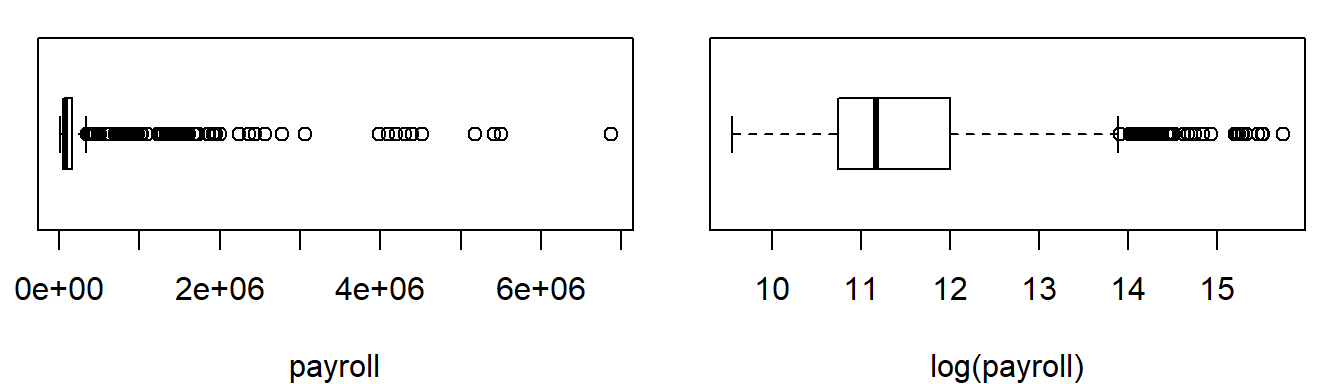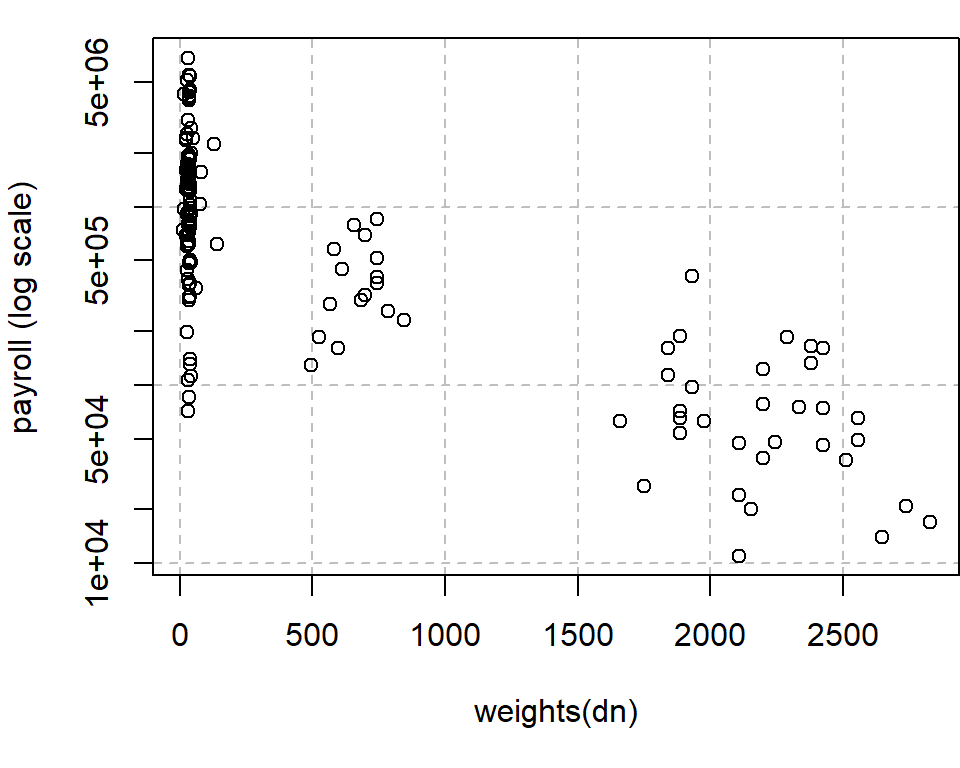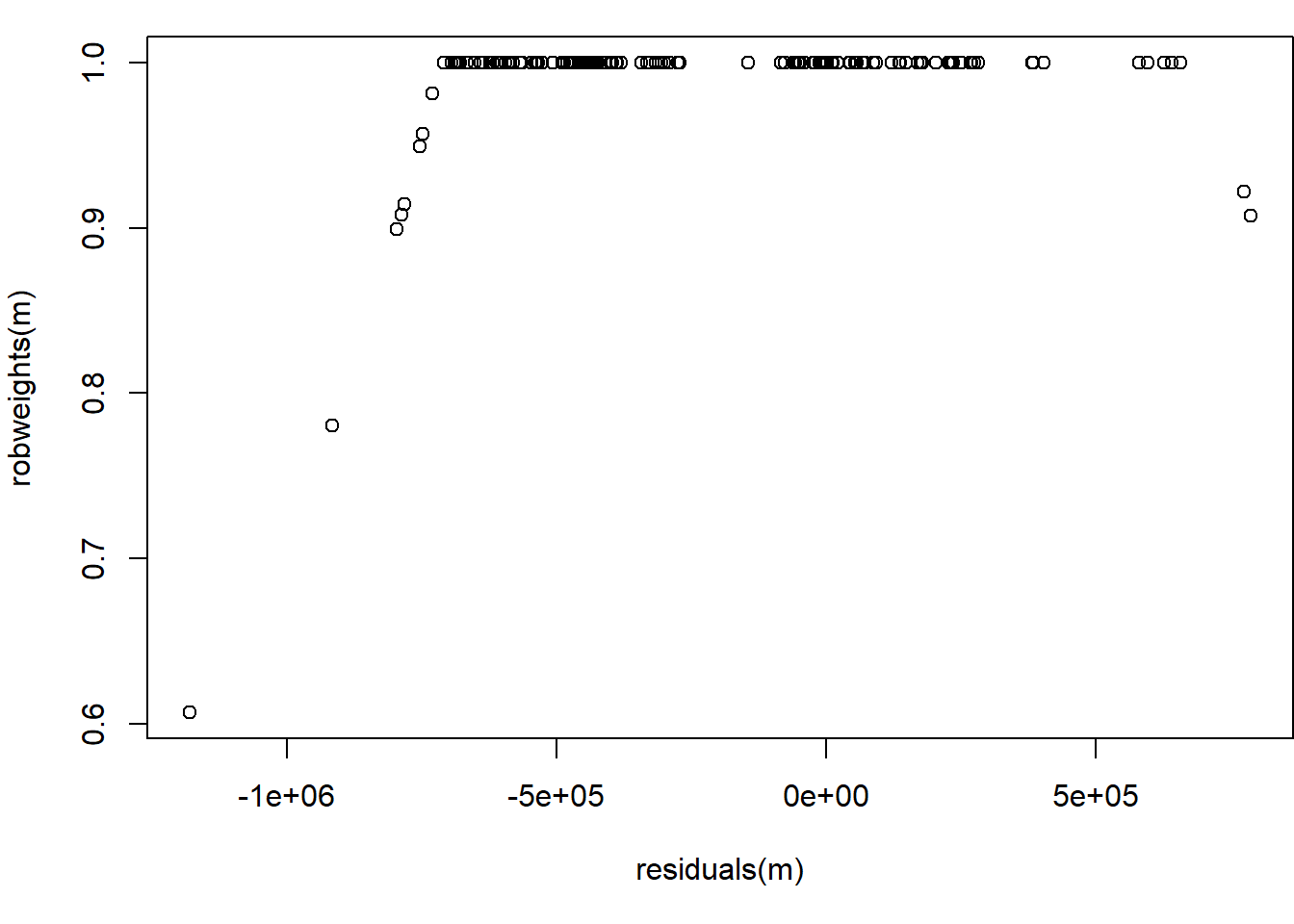## Outline

In this vignette, we discuss the robust Horvitz-Thompson (RHT) estimator of Hulliger (1995, 1999). The vignette is organized as follows.

• 1 Workplace data
• 2 Robust Horvitz-Thompson estimator
• References

Good to know.

The RHT estimating method is available in two “flavors”:

• bare-bone method
• survey method

Bare-bone methods are stripped-down versions of the survey methods in terms of functionality and informativeness. These functions may serve users and package developers as building blocks. In particular, bare-bone functions cannot compute variances.

> library("robsurvey", quietly = TRUE)

## 1. Workplace Data

The workplace sample consists of payroll data on $$n=142$$ workplaces or business establishments (with paid employees) in the retail sector of a Canadian province.

• The data display similar characteristics to the original 1999 Canadian Workplace and Employee Survey (WES), however, the workplace data are not those collected by Statistics Canada but have been generated by Fuller (2009, Example 3.1.1, Table 6.3).
• The sampling design is stratified by industry, geographic region, and size (size is defined using estimated employment). A sample of workplaces was then drawn independently in each stratum using simple random sample without replacement (sample size is determined by Neyman allocation).

The original weights of WES were about 2200 for the stratum of small workplaces, about 750 for medium-sized, and about 35 for large workspaces. Several strata containing very large workplaces were sampled exhaustively; see Patak et al. (1998).

> attach(workplace)

The variable of interest is payroll, and the goal is to estimate the population total of payroll in the retail sector.

> head(workplace, 3)
ID weight employment payroll strat   fpc
1  1    786         17  260000     2 10718
2  2     32        661 6873000     1  3432
3  3     36          3  366000     1  3432

### 1.1 Survey design object

For the survey methods (not bare-bone methods), we must load the survey package (Lumley, 2010, 2021)

> library("survey")

and specify a survey or sampling design object

> dn <- svydesign(ids = ~ID, strata = ~strat, fpc = ~fpc, weights = ~weight, data = workplace)

which appears (on print) with the following output in the console

Stratified Independent Sampling design
svydesign(ids = ~ID, strata = ~strat, fpc = ~fpc, weights = ~weight,
data = workplace)

### 1.2 Exploring the data

To get a first impression of the distribution of payroll, we examine two (design-weighted) boxplots of payroll (on raw and logarithmic scale). The boxplots are obtained using function survey::svyboxplot.From the boxplot with payroll on raw scale, we recognise that the sample distribution of payroll is skewed to the right; the boxplot on logarithmic scale demonstrates that log-transform is not sufficient to turn the skewed distribution into a symmetric distribution. The outliers need not be errors. Following Chambers (1986), we distinguish representative outliers from non-representative outliers ($$\rightarrow$$ see vignette “Basic Robust Estimators” for an introduction to the notion of non-/ representative outliers).

The outliers visible in the boxplot refer to a few large workplaces. Moreover, we assume that these outliers represent other workplaces in the population that are similar in value (i.e., representative outliers).

Detailed analysis.

We plotted the sampling weights against the logarithm of payroll.In the scatter plot, we can observe the following tendency:

• Large observations have rather small weights (and vice versa).
• Or, if we think of a linear regression fit, we would draw the line from the top left to the bottom right corner.

This tendency reflects the survey designers’ attempt to prevent influential outliers at the stage of designing the survey, that is, to prevent observations that are far from the bulk of values and have a large sampling weight. The survey designers achieved this by carefully sampling workplaces with large (anticipated) payroll with a sample inclusion probability equal or close to unity.

Although the designers did a great job, we are faced with the following problem: Because outliers have a sampling weight that is already close to unity, there is little we can do by reducing the sampling weight in order to limit the impact of an influential value. Hence, weight reduction ($$\rightarrow$$ see vignette “Basic Robust Estimators”) is not a productive strategy. Fortunately, this situation is where the RHT shines.

## 2 Robust Horvitz-Thompson Estimator

IMPORTANT

The RHT estimator is the method of choice for pps designs (i.e., designs without replacement where the sample inclusion probabilities are proportional to some measure of size). For equal-probability designs, the M-estimator of type = "rhj" (robust Hajek type estimator) tends to be superior; see vignette Basic Robust Estimators to learn more.

### 2.1 Bare-bone methods

The following bare-bone estimating methods are available:

• weighted_mean_huber()
• weighted_total_huber()
• weighted_mean_tukey()
• weighted_total_tukey()

The functions with postfix _tukey are M-estimators with the Tukey biweight $$\psi$$-function. The Huber RHT M-estimator of the payroll total can be computed with

> weighted_total_huber(payroll, weight, k = 8, type = "rht")
 15587090084

Note that we must specify type = "rht" for the RHT [the case type = "rhj" is discussed in the vignette “Basic Robust Estimators”]. Here, we have chosen the robustness tuning constant $$k = 8$$.

Good to know.

In general, the tuning constant k must be chosen larger than (loosely speaking) “we are used to choose it”. More precisely, in the context of an infinite population with a standard Gaussian distribution, the constant $$k = 1.345$$ ensures that the Huber $$M$$-estimator of location achieves 95% efficiency compared with the arithmetic mean under the Gaussian model. The efficiency considerations underlying the choice of $$k = 1.345$$ do not carry over to distributions other than the Gaussian.

All bare-bone methods can be called with the argument info = TRUE (default: FALSE). This instructs the functions to return a list with estimate-specific information $$\rightarrow$$ see vignette “Basic Robust Estimators” to learn more.

The Huber M-estimator can be computed for an asymmetric Huber $$\psi$$-function by calling the function with the additional argument asym = TRUE.

The M-estimators are computed by iterative methods. If the algorithm fails to converge, the functions return NA. By default, the algorithm uses a maximum of maxit = 50 iterations and a numerical tolerance criterion of tol = 1e-5 as a stopping rule. Other values of maxit and tol can be specified in the function call; see svyreg_control().

### 2.2 Survey methods

The following survey method are available;

• svymean_huber()
• svytotal_huber()
• svymean_tukey()
• svytotal_tukey()

The survey method of the RHT (and its standard error) is

> m <- svytotal_huber(~payroll, dn, k = 8, type = "rht")
> m
total        SE
payroll 1.559e+10 1.198e+09

The summary() method summarizes the most important facts about the estimate.

> summary(m)
Huber M-estimator (type = rht) of the sample total

total        SE
payroll 1.559e+10 1.198e+09

Robustness:
Psi-function: with k = 8
mean of robustness weights: 0.9917

Algorithm performance:
converged in 4 iterations
with residual scale (weighted MAD): 89474

Sampling design:
Stratified Independent Sampling design
svydesign(ids = ~ID, strata = ~strat, fpc = ~fpc, weights = ~weight,
data = workplace)

The estimated location, variance, and standard error can be extracted from object m with the following commands.

> coef(m)
payroll
15587090084
> vcov(m)
Variance
payroll 1.434857e+18
> SE(m)
 1197855270

For M-estimators, the estimated scale (weighted MAD) can be extracted with the scale() function.

> scale(m)
 89474.01

• residuals() to extract the residuals
• fitted() to extract the fitted values under the model in use
• robweights() to extract the robustness weights

In the following figure, the robustness weights of object m are plotted against the residuals. The Huber RHT M-estimator downweights observations at both ends of the residuals’ distribution.

> plot(residuals(m), robweights(m))An adaptive M-estimator of the total (or mean) is defined by letting the data chose the tuning constant $$k$$. This approach is available for the RHT estimator $$\rightarrow$$ see vignette “Basic Robust Estimators”, Chap. 5.3 on M-estimators.

## References

CHAMBERS, R. (1986). Outlier Robust Finite Population Estimation. Journal of the American Statistical Association 81, 1063–1069, DOI: 10.1080/01621459.1986.10478374.

FULLER, W. A. (2009). Sampling Statistics, Hoboken (NJ): John Wiley and Sons, DOI: 10.1002/9780470523551.

HULLIGER, B. (1995). Outlier Robust Horvitz–Thompson Estimators. Survey Methodology 21, 79–87.

HULLIGER, B. (1999). Simple and robust estimators for sampling, in: Proceedings of the Survey Research Methods Section, American Statistical Association, pp. 54–63.

HULLIGER, B. (2006). Horvitz–Thompson Estimators, Robustified. In: Encyclopedia of Statistical Sciences ed. by Kotz, S. Volume 5, Hoboken (NJ): John Wiley and Sons, 2nd edition, DOI: 10.1002/0471667196.ess1066.pub2.

LUMLEY, T. (2010). Complex Surveys: A Guide to Analysis Using R: A Guide to Analysis Using R, Hoboken (NJ): John Wiley and Sons.

LUMLEY, T. (2021). survey: analysis of complex survey samples. R package version 4.0, URL https://CRAN.R-project.org/package=survey.